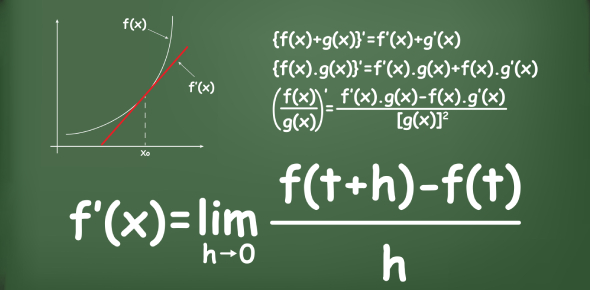# Derivatives Of Exponential And Logarithmic Functions Quiz Questions

10 Questions | Attempts: 686
ShareSettingsTake up this quiz to review your knowledge of derivatives of exponential and logarithmic functions by choosing suitable answers to the questions asked here. The logarithmic function is the inverse of the exponential function. If you think your calculus is really strong and can score well on the test, why don't you clear out your doubt now? Also, the quiz will check your differentiating skills. Shall we start the quiz then? Give your best!

• 1.
Differentiate y= 3e-4x
• A.

Dy/dx = -3e-3x

• B.

Dy/dx= -12e4x

• C.

Dy/dx= -12e-4x

• D.

Dy/dx = 34e4x

• 2.
Is differentiation of e5x-1 is 5e5x-1 true or false?
• A.

True

• B.

False

• 3.
Differentiate y= 2e5x+2
• A.

Dy/dx= -10e5x

• B.

Dy/dx= 10e5x+2

• C.

Dy/dx= 2e5x

• D.

Dy/dx= e5x+2

• 4.
Differentiate y= e2-3x
• A.

Dy/dx= 3e

• B.

Dy/dx= 3e2-3x

• C.

Dy/dx= 2e2-3x

• D.

Dy/dx= -3e2-3x

• 5.
Is differentiation of e1+5x is -5e1+5x true or false?
• A.

True

• B.

False

• 6.
Differentiate y= ln(7x+2)
• A.

Dy/dx = 7 /(7x+2)

• B.

Dy/dx= 2/(7x+2)

• C.

Dy/dx= 7(7x+2)

• D.

Dy/dx= 7x+2

• 7.
Is Differentiation of ln(5x) is 1/x true or false?
• A.

True

• B.

False

• 8.
Differentiate y =ln(x-8)
• A.

Dy/dx= 1

• B.

Dy/dx = 1/(x-8)

• C.

Dy/dx= 8/(x-8)

• D.

Dy/dx= -1/(x-8)

• 9.
Is differentiation of ln(4x2-1) is 4x/(4x2-1) true or false?
• A.

True

• B.

False

• 10.
Differentiate y= ln(2+3x2)
• A.

Dy/dx= 6x

• B.

Dy/dx= 3x/(2+3x)

• C.

Dy/dx= 2/(2+3x2)

• D.

Dy/dx= 6x/(2+3x2)

## Related TopicsBack to top
×

Wait!
Here's an interesting quiz for you.